In OfficeCentral, you can configure the system to suit OfficeCentral with your company’s salary calculation policy. In this module, you can also add employees’ bank, country’s configuration and approver.

1. SETTINGS

In Payroll module, you can set salary regulation based on country. This is because OfficeCentral containssalary calculation for 2 countries, Malaysia and Indonesia.

For settings:

1. Go to “Payroll” section, then go to “Configurations”, next go to “Settings” and click on“Settings”.2. Here you can view “Payroll Country” available. Once setting is done please click on “Save
Changes”.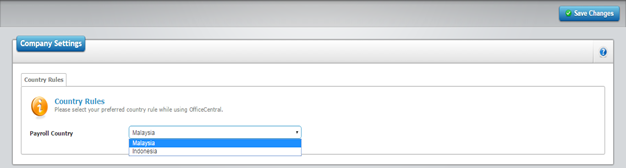2. PAYROLL GROUPS

In OfficeCentral, you can add several payroll groups if you have different ways of calculating salary for your
staff. If salary calculation for each staff is the same, you can key in only one group in this section.

1. To access this module, you need to go to “Payroll”, select “Payroll Groups” and next “View List”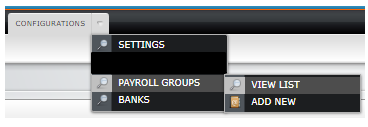2. Follow is the screen display: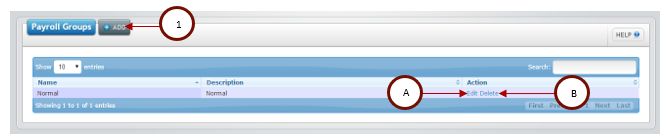Picture 6.7: List of Payroll group in your company

Description:

 Number Description 1 Click on “Add” to add new type of payroll group. A Click on “Edit” to carry out any modification on available data. B Click on “Delete” to erase the existing payroll group. However, the data can only be erased if it is yet to be used. Please take note that an erased data cannot be retrieved.

1. Click on “Add” button as per the above picture.

2. You will be able to view the next screen display as follow:Description:

NumberDescription
AEnter the group name. For example, “Standard”.
BEnter the description for the group name.
CSelect salary calculation type.
Picture 6.9: Selections for salary calculation type
 Fixed number of days per month Select this option if you are calculating the salary based on fixed number of days monthly, for example 26 days.By selecting this option, the calculation of 1 day unpaid leave is 1/(number of days) * basic salary. Therefore, if the number of days is 26 and basic salary is RM2000/month, calculation for 1 day unpaid leave is as follow: 1/26*2000.Overtime calculation will also follow the same rate, that is 1 day salary will be calculated as 1/26*2000. Based on total number of days in the month Select this option if you are calculating salary based on number of days of that particular month. For instance, June will be calculated as 30 days while July will be calculated as 31 days.By selecting this option, the calculation for 1 day unpaid leave is 1/(number of days in that month) * basic salary. Therefore, if that month is the month of June which has 30 days and basic salary is RM2000/month, calculation for 1 day unpaid leave is: 1/30*2000.Calculation for overtime will also follow the same rate that is 1 working day will be calculated as 1/30*2000. Based on total number of days between cutoff Select this option if you are calculating salary base on number of days between “Start Date” and “Cutoff Date”.By selecting this option, the calculation for 1 day unpaid leave will be based on 1/(number of days between the 2 dates) * basic salary.For instance, if you choose “27 August 2015” as the start date and “26 September 2015” as the cutoff date, system will calculate it as 31 days. If the basic salary is RM2000/month, calculation for 1 day unpaid leave is: 1/31*2000.Overtime calculation will also follow the same rate, that is 1 working day will be calculated as 1/31*2000. Based on 5 working days (Monday-Friday) per week in a month Select this option if you are calculating salary based on number of working days in a month with 5 working days (Monday - Friday) weekly.By selecting this option, 1 day unpaid leave will be calculated based on 1/(number of working days in that month)*basic salary.For example, if you are calculating for the month of August 2015, system will count as 21 days as there are 21 working days (Monday - Friday) in August 2015. If the basic salary is RM2000/month, 1 day unpaid leave calculation is: 1/21*2000.Overtime calculation will also follow the same rate, that is 1 working day is calculate as 1/21*2000. Based on 5 working days (Monday-Friday) per week between cutoff dates This option is for salary calculation based on number of working days between 2 dates (Start date and Cutoff Date) with 5 working days (Monday - Friday) in a week.By selecting this option, 1 day unpaid leave will be calculated based on 1/(number of working days between the 2 dates)*basic salary. For instance, if you choose “27 August 2015” as the start date and “26 September 2015” as the cutoff date, system will count as 22 days. If the basic salary is RM2000/month, 1 unpaid leave calculation will be: 1/22*2000.Overtime calculation will also follow the same rate, that is 1 working day calculation is 1/22 * 2000. Based on 5.5 working days (Mon-Fri, Half-day on Saturdays) per week in a month. Select this option if you are calculating salary based on number of working days in a month with 5.5 working days (Monday - Friday, half day on Saturday) in a week.By selecting this option, 1 day unpaid leave will be calculated based on 1/(number of working days in that month)*basic salary.For example, if you are calculating for the month of August 2015, system will count as 23 days as there are 23 working days (Monday - Friday, half day on Saturday) in August 2015. If the basic salary is RM2000/month, 1 day unpaid leave calculation is: 1/23*2000.Overtime calculation will also follow the same rate, that is 1 working day is calculate as 1/23*2000. Based on 5.5 working days (Mon-Fri, Half-day on Saturdays) per week between start date and cutoff date. This option is for salary calculation based on number of working days between 2 dates (Start date and Cutoff Date) with 5.5 working days (Monday - Friday, half day on Saturday) in a week.By selecting this option, 1 day unpaid leave will be calculated based on 1/(number of working days between the 2 dates)*basic salary. For instance, if you choose “27 August 2015” as the start date and “26 September 2015” as the cutoff date, system will count as 25 days. If the basic salary is RM2000/month, 1 unpaid leave calculation will be: 1/25*2000.Overtime calculation will also follow the same rate, that is 1 working day calculation is 1/25 * 2000. Based on 6 working days(Mon-Saturday) per week in a month. Select this option if you are calculating salary based on number of working days in a month with 5 working days (Monday - Saturday) in a week.By selecting this option, 1 day unpaid leave will be calculated based on 1/(number of working days in that month)*basic salary.For example, if you are calculating for the month of August 2015, system will count as 25 days as there are 25 working days (Monday - Saturday) in August 2015. If the basic salary is RM2000/month, 1 day unpaid leave calculation is: 1/25*2000.Overtime calculation will also follow the same rate, that is 1 working day is calculate as 1/25*2000. Based on 6 working days (Mon-Saturday) per week between start date and cutoff date. This option is for salary calculation based on number of working days between 2 dates (Start date and Cutoff Date) with 6 working days (Monday - Saturday) in a week.By selecting this option, 1 day unpaid leave will be calculated based on 1/(number of working days between the 2 dates)*basic salary. For instance, if you choose “27 August 2015” as the start date and “26 September 2015” as the cutoff date, system will count as 27 days. If the basic salary is RM2000/month, 1 unpaid leave calculation will be: 1/27*2000.Overtime calculation will also follow the same rate, that is 1 working day calculation is 1/27 * 2000. Based on staff’s location’s working days in a month This option is using the same calculation above in the month of salary calculation but following to working days set at a particular location. For example, in Kelantan, the working day is Sunday - Thursday. Therefore, for this location it is recommended to use this option for salary calculation (if not using the fixed number of days as in the first and second option). Based on staff’s location’s working days between cutoff This option is using the same calculation above that is the calculation based on number of working days between 2 dates (start date and cutoff date) but following the working days set at a particular location. For example, in Kelantan, the working day is Sunday - Thursday. Therefore, for this state, it is recommended to select this option for salary calculation (if not using the fixed number of days as in the first and second option).
DEnter the number of working days in a month. This option is prepared should user choose to set a fixed number of days, for example 26 days in a month.
EEnter the number of working hours in a day. This information is use to calculate overtime if any.

Here you can configure the settings for overtime. Please be reminded that this setting is only for overtime rate using multiplier only.Description:

 A Key in the overtime multiplier for each hour worked after working hour. For example, if overtime salary given is the same as working hour, key in 1 in the box. If the company gives 1.5 times, key in 1.5 in the box. B Key in overtime multipliers for each hour worked on rest day such as Sunday. For instance, if the overtime salary given is the same as normal working hour, key in 1 in the box. If the company gives twice as much, key in the number 2 in the box. C Key in overtime multipliers for each hour worked on public holiday such as Merdeka Day holiday on 31 August 2015. For instance, if the overtime salary given is the same as normal working hour, key in 1 in the box. If the company gives triple, key in the number 3 in the box. D Key in overtime multipliers for each hour worked after normal working hours. For example, if the overtime salary given is the same as normal working hour, key in 1 in the box. If the company gives1.5 times, key in 1.5 in the box. E Key in overtime multipliers for each hour worked on rest day such as Sunday. For instance, if the overtime salary given is the same as normal working hour, key in 1 in the box. If the company gives twice as much, key in the number 2 in the box. F Key in overtime multipliers for each hour worked on public holiday such as Merdeka Day Holiday on 31 August 2015. For instance, if the overtime salary given is the same as normal working hour, key in 1 in the box. If the company gives triple, key in the number 3 in the box.

If you want to fix an amount for overtime, you can tick “√” in the “Use Fixed Value for Overtime” box.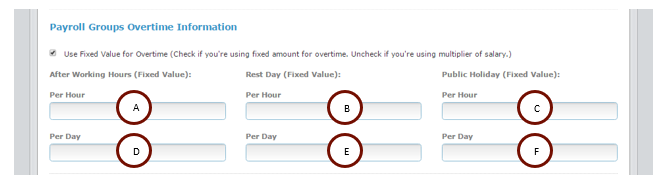Description:

 A Key in the overtime multiplier for each hour worked after working hour. For example, if overtime salary given is the same as working hour, key in 1 in the box. If the company gives 1.5 times, key in 1.5 in the box. B Key in overtime multipliers for each hour worked on rest day such as Sunday. For instance, if the overtime salary given is the same as normal working hour, key in 1 in the box. If the company gives twice as much, key in the number 2 in the box. C Key in overtime multipliers for each hour worked on public holiday such as Merdeka Day holiday on 31 August 2015. For instance, if the overtime salary given is the same as normal working hour, key in 1 in the box. If the company gives triple, key in the number 3 in the box. D Key in overtime multipliers for each hour worked after normal working hours. For example, if the overtime salary given is the same as normal working hour, key in 1 in the box. If the company gives1.5 times, key in 1.5 in the box. E Key in overtime multipliers for each hour worked on rest day such as Sunday. For instance, if the overtime salary given is the same as normal working hour, key in 1 in the box. If the company gives twice as much, key in the number 2 in the box. F Key in overtime multipliers for each hour worked on public holiday such as Merdeka Day Holiday on 31 August 2015. For instance, if the overtime salary given is the same as normal working hour, key in 1 in the box. If the company gives triple, key in the number 3 in the box.Here you can configure the settings whether the overtime payment needs to be contributed to statutory bodies such as EPF, KWAP, SOCSO, Employee Insurance System, Income Tax, Zakat and PSMB. If the payment need to be contributed, you will need to check “√” in the respective box.

3. BANK

In this module, you can add banks that are available in your company. These banks are your bank and banks
used by your employee for salary payment.

1. To access this page, go to PAYROLL, select CONFIGURATIONS, and click on BANKS.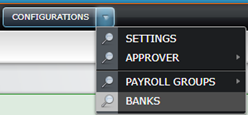2. After that you will be see the following screen.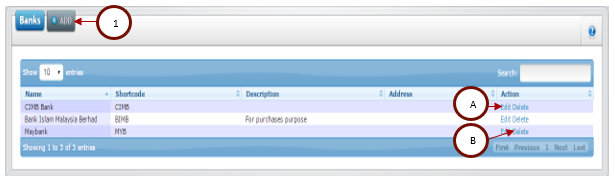Description:

 Number Description 1 Click “Add” button to add new bank. A Click “Edit” button to carry out any modification to the existing bank. B Click on “Delete” to erase existing bank. However, bank can only be deleted if it is yet to be used. Please take note that erased data cannot be retrieve.

1. Fill in the required information on the page to add new bank that is not available in the system.Description:

 Number Description A Bank Name. B Abbreviation for the bank. For instance, Maybank is usually referred to as “MBB”. C Bank’s account number D Bank’s address if the company has an account with the bank. E Explanation on the bank if any. If not, please leave the space empty.

2. Once done, click on “Save” on the top right of the page. The bank will be added to the list of banks.